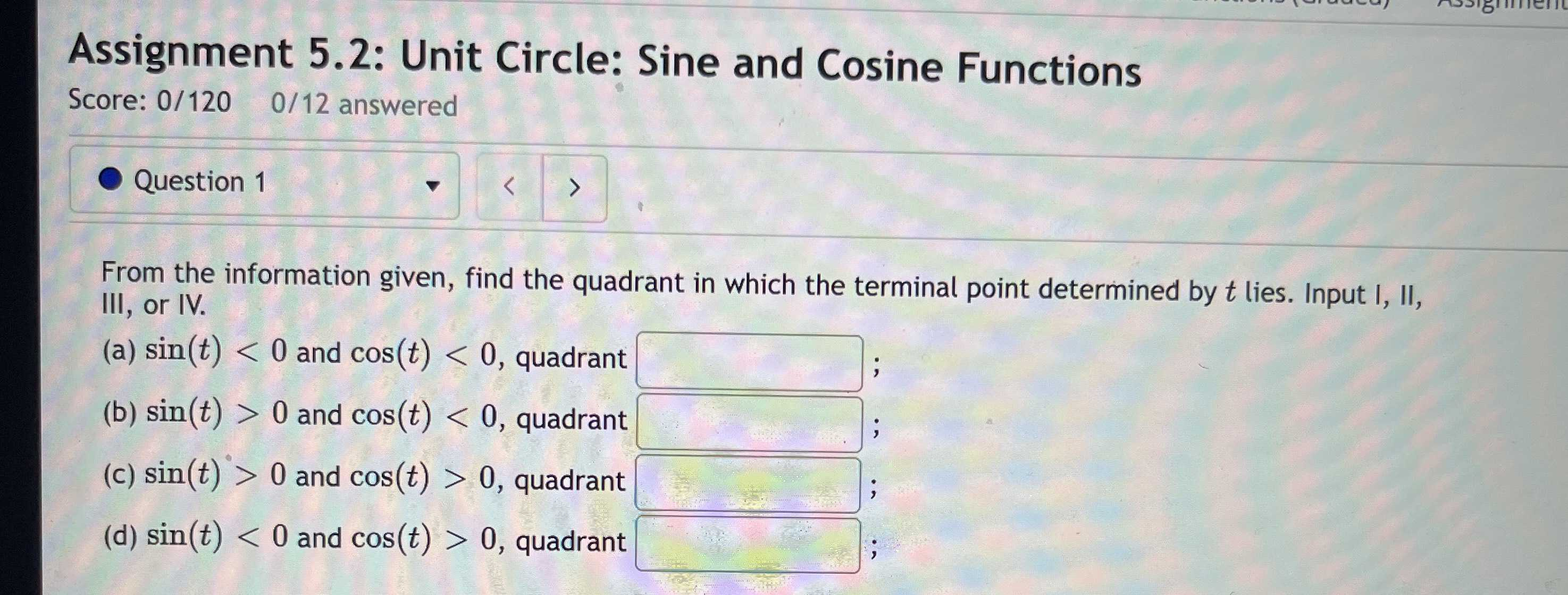### Still have math questions?

Trigonometry
QuestionFrom the information given, find the quadrant in which the terminal point determined by $$t$$ lies. Input I, II, III, or IV.

(a) $$\sin ( t ) < 0$$ and $$\cos ( t ) < 0 ,$$ quadrant

(b) $$\sin ( t ) > 0$$ and $$\cos ( t ) < 0 ,$$ quadrant

(c) $$\sin ( t ) > 0$$ and $$\cos ( t ) > 0 ,$$ quadrant

(d) $$\sin ( t ) < 0$$ and $$\cos ( t ) > 0 ,$$ quadrant